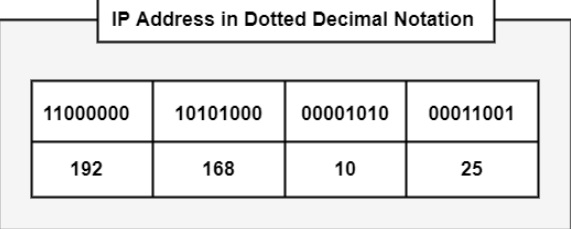IPv4 addresses are merely used as identifiers, which operate at the network layer to identify IP packets source or destination. The version of IP that is in use is known as IPv4. In this version, every node on the Internet can have one or more interfaces, and we are needed to recognize each of these devices with a specific address assigned to each of them. It represents that each node is created one or more IP addresses to conjure TCP/IP. These are logical addresses and include 32 bits.

IP addresses are defined using binary notation with a 32-bit long string. Dotted decimal notations are used to make strings easily, in which periods or dots separate four decimal numbers from 0 to 255, describing 32 bits.

For example, the IPv4 address 11000000101010000000101000011001 is represented as 192.168.10.25 in dotted decimal notation.

Following are the steps to convert an IPv4 address from binary notation to dotted-decimal notation−

• Break 32-bit long address into segments of 8-bit blocks: 11000000 10101000 00001010 00011001
• Write decimal similar to each structure: 192 168 10 25
• Divide the blocks with periods: 192.168.10.25

The figure shows the IP address structure.## Dotted Decimal Notation

We have seen that the IPv4 address is expressed as a 32-bit number in dotted decimal notation. IP addresses may have a fixed part and variable part depending upon the allocation of total addresses to you or your organization.

The fixed part of the address may be from one octet to three octets, and the remaining octets will then be available for the variable part.

For example, you can take an IP address like 192.168.10.25. Now set all constant bits to 1 and set all variable bits to 0. This gives 11111111 11111111 00000000 00000000. On converting it in dotted-decimal notation, the outcome is 255.255.0.0.

This dotted-decimal notation with constant and variable methods can address prefixes to 192.168.10.25 and is represented as 192.168.10.25, 255.255.0.0. This method of expressing the prefix length as a dotted-decimal number is known as a network mask or subnet mask notation.

Updated on: 05-May-2021

2K+ Views